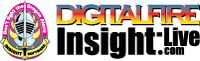•The secret to cool bodies and glazes is a lot of testing.
•The secret to know what to test is material and chemistry knowledge.
•The secret to learning from testing is documentation.
•The place to test, do the chemistry and document is an account at https://insight-live.com
•The place to get the knowledge is https://digitalfire.com

Sign-up at https://insight-live.com today.

Ravenscrag Slip 1000F Roast

Silty fusible low iron clay

 Oxide Analysis Formula BaO 0.30% 0.007 CaO 10.70% 0.683 MgO 1.80% 0.160 K2O 3.20% 0.122 Na2O 0.50% 0.029 TiO2 0.40% 0.018 Al2O3 13.40% 0.470 SiO2 65.80% 3.919 Fe2O3 0.90% 0.020 LOI 3.00 Oxide Weight 347.19 Formula Weight 357.93

XML for Import into INSIGHT

<?xml version="1.0" encoding="UTF-8"?> <material name="Ravenscrag Slip 1000F Roast" descrip="Silty fusible low iron clay" searchkey="" loi="0.00" casnumber=""> <oxides> <oxide symbol="BaO" name="Barium Oxide, Baria" status="" percent="0.300" tolerance=""/> <oxide symbol="CaO" name="Calcium Oxide, Calcia" status="" percent="10.700" tolerance=""/> <oxide symbol="MgO" name="Magnesium Oxide, Magnesia" status="" percent="1.800" tolerance=""/> <oxide symbol="K2O" name="Potassium Oxide" status="" percent="3.200" tolerance=""/> <oxide symbol="Na2O" name="Sodium Oxide, Soda" status="" percent="0.500" tolerance=""/> <oxide symbol="TiO2" name="Titanium Dioxide, Titania" status="" percent="0.400" tolerance=""/> <oxide symbol="Al2O3" name="Aluminum Oxide, Alumina" status="" percent="13.400" tolerance=""/> <oxide symbol="SiO2" name="Silicon Dioxide, Silica" status="" percent="65.800" tolerance=""/> <oxide symbol="Fe2O3" name="Iron Oxide, Ferric Oxide" status="" percent="0.900" tolerance=""/> </oxides> <volatiles> <volatile symbol="LOI" name="Loss on Ignition" percent="3.000" tolerance=""/> </volatiles> </material>